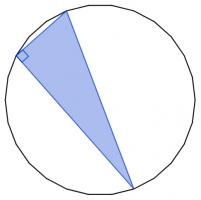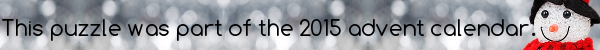mscroggs.co.uk
mscroggs.co.uksubscribe

# Puzzles

## Archive

Show me a random puzzle
▼ show ▼

## 23 DecemberToday's number is the area of the largest area rectangle with perimeter 46 and whose sides are all integer length.

## 12 DecemberThese three vertices form a right angled triangle.
There are 2600 different ways to pick three vertices of a regular 26-sided shape. Sometime the three vertices you pick form a right angled triangle.
Today's number is the number of different ways to pick three vertices of a regular 26-sided shape so that the three vertices make a right angled triangle.

## Equal lengths

The picture below shows two copies of the same rectangle with red and blue lines. The blue line visits the midpoint of the opposite side. The lengths shown in red and blue are of equal length.
What is the ratio of the sides of the rectangle?

## Is it equilateral?

In the diagram below, $$ABDC$$ is a square. Angles $$ACE$$ and $$BDE$$ are both 75°.
Is triangle $$ABE$$ equilateral? Why/why not?

## Two semicircles

The diagram shows two semicircles.
$$CD$$ is a chord of the larger circle and is parallel to $$AB$$. The length of $$CD$$ is 8m. What is the area of the shaded region (in terms of $$\pi$$)?

## 1 DecemberWhat is area of the largest area rectangle which will fit in a circle of radius 10?

## Cutting corners

The diagram below shows a triangle $$ABC$$. The line $$CE$$ is perpendicular to $$AB$$ and the line $$AD$$ is perpedicular to $$BC$$.
The side $$AC$$ is 6.5cm long and the lines $$CE$$ and $$AD$$ are 5.6cm and 6.0cm respectively.
How long are the other two sides of the triangle?

## Quarter circle

Source: Maths Jam
A quarter circle is drawn in a square. A rectangle is drawn in the corner of the square which touches the circle and has sides of length 8 and 1.
What is the length of a side of the square?
© Matthew Scroggs 2018